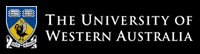School of Computer Science and Software Engineering

# School of Computer Science and Software Engineering

## CITS4406 Problem Solving and Programming

#### Labsheet 5: Text Formatting

This labsheet is in two parts: a number of review exercises taken from the text that reinforce concepts from the lectures; and an important problem-solving technique, motivated by its use in solving a larger-sized problem representative of the types of problems that can be solved with computers.

#### Review Exercises (Zelle, Chapter 5 and Lecture 11)

1. Show the string that would result from each of the following string formatting operations. If
the operation is not legal, explain why.

(a) "Looks like {1} and {0} for breakfast".format("eggs", "spam")

(b) "There is {0} {1} {2} {3}".format(1,"spam", 4, "you")

(c) "Hello {0}".format("Susan", "Computewell")

(d) "{0:0.2f} {0:0.2f}".format(2.3, 2.3468)

(e) "{7.5f} {7.5f}".format(2.3, 2.3468)

(f) "Time left {0:02}:{1:05.2f}".format(1, 37.374)

(g) "{1:3}".format("14")

2. Write a function that generates a list of all prime numbers upto N. The function should take N as parameter and return the list.

3. Write a function that returns a list of the first N prime numbers.

Hint for 2 and 3: You can skip testing even numbers as no even number besides 2 is a prime number. Also notice that your function must "return a list".

4. You want to draw 2 circles such that they do not intersect. Write a function that takes input from the user i.e. center, radius of the two circles and then calls another function to check if the circles intersect.

Hint: Calculate the distance between the center of the circles.

5. Write a function that takes a list as a parameter and swaps pairs of consecutive values in the list i.e. index 0 and 1, 2 and 3, 4 and 5 and so on. If there are odd number of elements in the list, the last element remains the same.

6.Write a function that reads a text file, encrypts the text with public key encryption and writes the encrypted text to another file.

Hint: Use the public, private key pairs provided in lecture 11 for question 6 and 7.

7.Write another function that will read the encrypted file, decypher it and print the plain text on the screen.

#### Problem Solving Techniques: Enumeration and Search

Here is a string that was encoded by a Caesar cipher with an unknown distance value:

"T! x\$r&'}r&z! %21j!'1~zxy&1"r%%1TZedBEAB?"

The text can contain any of the printable ASCII characters (32-126, for our purposes). Implement an algorithm for cracking this code.

The problem solving technique of Search, or Enumeration, is simply the idea of systematically trying a range of possible answers to see which one is the actual answer. This is useful if you have some way of generating a complete list of possible answers, and some way of recognizing the right answer from amongst those on the list. This is particularly useful if you have a computer that can do all the hard and boring work.

Use Enumeration to solve the following "Cryptarithmetic" Puzzle. In the sum SEND + MORE = MONEY, each letter stands for one (and only one digit) 0,...,9. Furthermore, none of the three numbers starts with the digit 0. Work out which digit each letter stands for.

# The University of Western Australia

## University information

CRICOS Code: 00126G